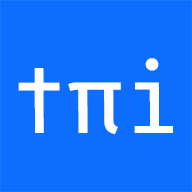# What is the maximum length of the multiplication of two integers?

Posted on
Authors
•Name
ansidev
@ansidev

## Question

Given two non-negative integers `m1` and `n1`, `m1` has `m` digits and `n1` has `n` digits. `p1` is the product of `m1` and `n1` (`p1 = m1 x n1`), `p1` has `p` digits.

What is the maximum value of `p`?

• 0 ≤ m1 ≤ 10m - 1
• 0 ≤ n1 ≤ 10n - 1
• 10k - 1 is an integer has k digits of 9
``````10^k - 1 = 9.....9
|_____|
k digits of 9
10^k - 1 = 9.....9
|_____|
k digits of 9
``````

p1 = m1 x n1 ≤ (10m -1) x (10n -1)
= 10m+n - 10m - 10m + 1
= 10m+n - 1 - (10m - 1) - (10m - 1)
≤ 10m+n - 1

So, p1 can have maximum `m + n` digits.